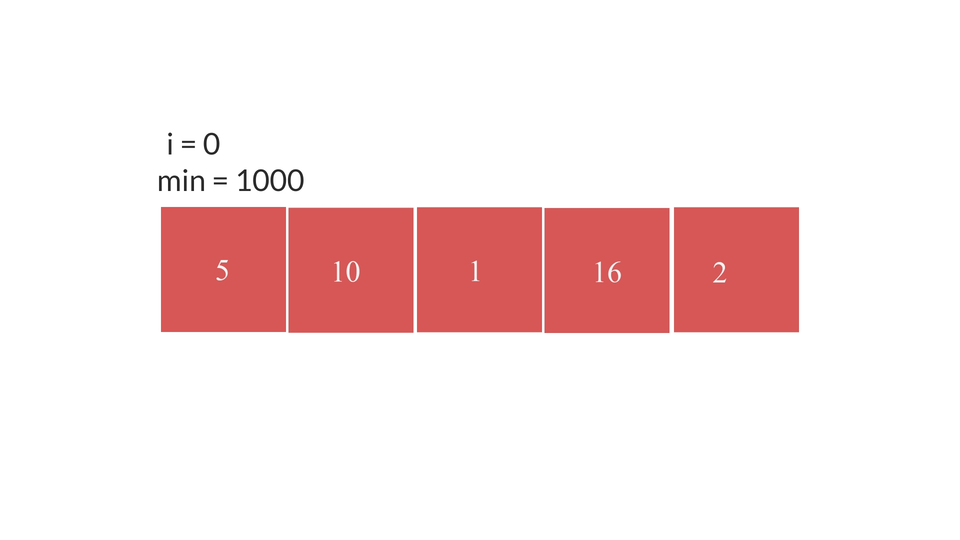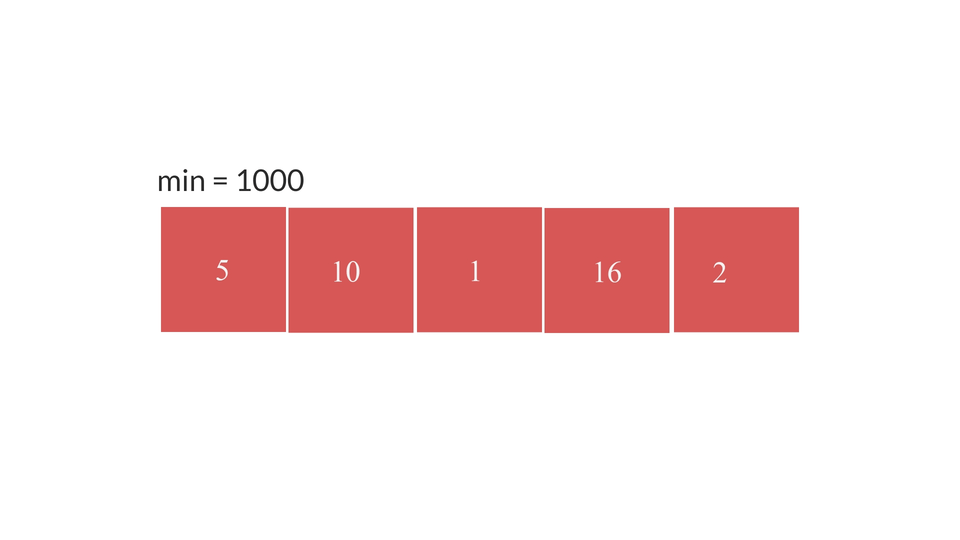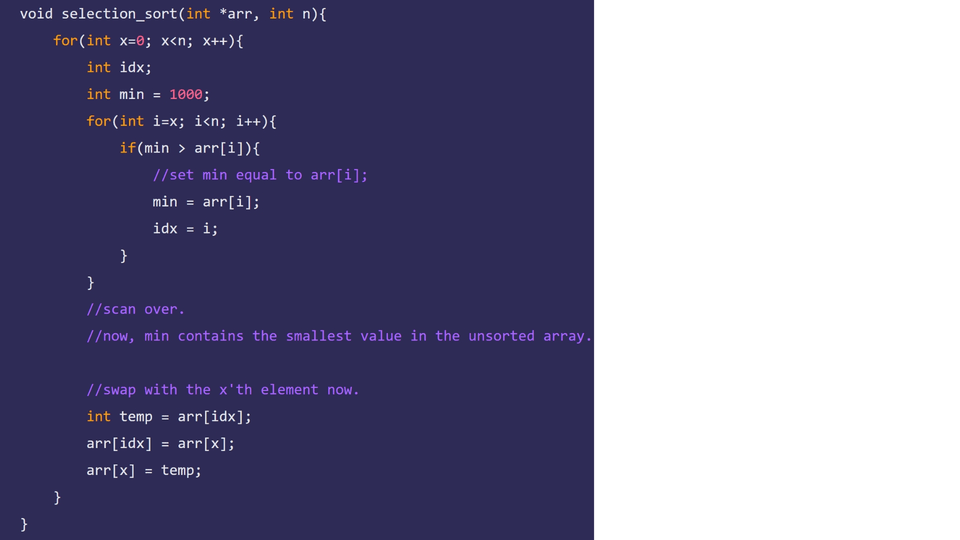# Selection SortCreated September 10, 2021

In this article I'm gonna talk about selection sort algorithm and how to implement it in C language.

## What is Selection Sort?

The main idea behind this sorting algorithm is to scan the unsorted part of the array repeatedly to find the smallest element in it and placing it at the beginning of this unsorted array.

You have to Select smallest element in the array after scanning the whole unsorted array. That's why it is called Selection Sort.

## Algorithm

The main concept is -

1. scanning the unsorted array to find the smallest element.
2. placing the smallest element at the beginning of the array.

### 1. Scanning for the smallest element

1. set min equal to some huge value, let's say 1000 for this example.
2. Iterate over the whole unsorted part of the array(i ranging from 0 to length of array-1).
3. in each iteration check if min > i'th element.
4. if so, set min equal to the i'th element.
5. else, do not do anything.### 2. Placing at the beginning of the sorted array

1. set x = 0.
2. create a for-loop for x from 0 to n-1
3. scan the array for the smallest element.
4. swap the smallest element with the x'th element.
5. as per the for-loop statement, repeat the 3 and 4 th steps until x == n-1.## The code

The code for selection sort looks something like this -

``````void selection_sort(int *arr, int n){
for(int x=0; x<n; x++){
int idx;
int min = 1000;
for(int i=x; i<n; i++){
if(min > arr[i]){
//set min equal to arr[i];
min = arr[i];
idx = i;
}
}
//scan over.
//now, min contains the smallest value in the unsorted array.

//swap with the x'th element now.
int temp = arr[idx];
arr[idx] = arr[x];
arr[x] = temp;
}
}
``````

The whole code is -

``````#include <stdio.h>

void selection_sort(int *arr, int n){
for(int x=0; x<n; x++){
int idx;
int min = 1000;
for(int i=x; i<n; i++){
if(min > arr[i]){
//set min equal to arr[i];
min = arr[i];
idx = i;
}
}
//scan over.
//now, min contains the smallest value in the unsorted array.

//swap with the x'th element now.
int temp = arr[idx];
arr[idx] = arr[x];
arr[x] = temp;
}

//print the array.
for(int i=0; i<n; i++){
printf("%d ", arr[i]);
}printf("\n");
}

int main(){
int n;
printf("Enter number of elements: ");
scanf(" %d", &n);

int arr[n];
for(int i=0; i<n; i++){
printf("Enter element: ");
scanf(" %d", &arr[i]);
}

//sort the array;
selection_sort(arr, n);
}
``````

## Time complexitySo, like bubble sort the time complexity of this algorithm is also O(n²). Both the worst case and best case have O(n²) time complexity. As the number of swaps in this algorithm is comparatively less than that in bubble sort, it considered to be more efficient than bubble sort.